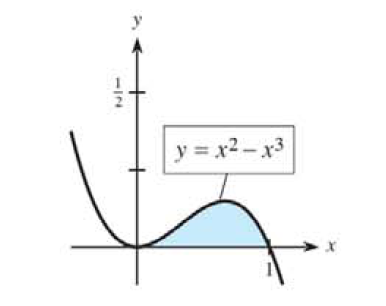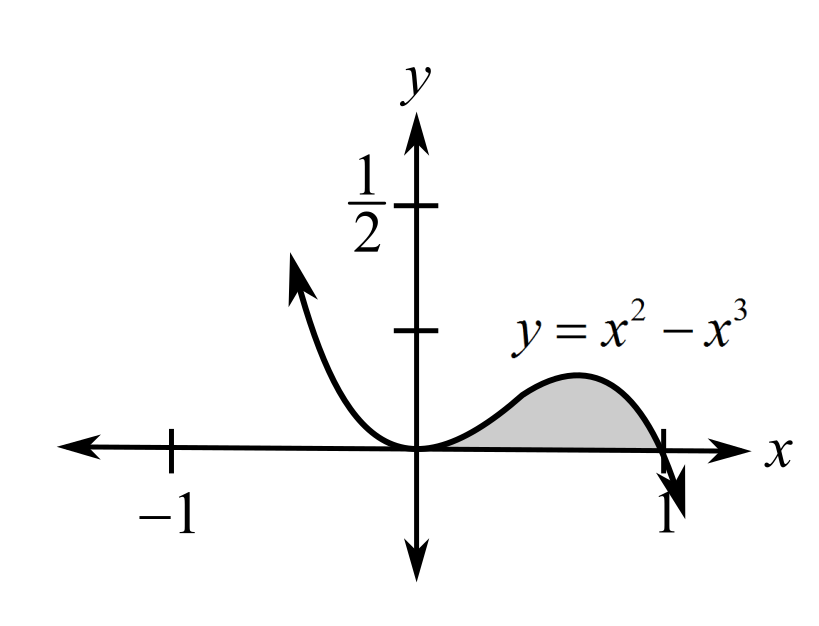Chapter 13.2, Problem 40E### Mathematical Applications for the ...

11th Edition
Ronald J. Harshbarger + 1 other
ISBN: 9781305108042

#### Solutions

Chapter
Section### Mathematical Applications for the ...

11th Edition
Ronald J. Harshbarger + 1 other
ISBN: 9781305108042
Textbook Problem

# In Problems 39-42, (a) write the integral that describes the area of the shaded region and (b) find the area.(a)

To determine

To calculate: The integral that describes the area of the shaded region in the figure,Explanation

Given Information:

The provided figure is,

Formula used:

The area under a curve from a to b is the absolute value of the definite integral of the function calculated with the lower limit a and upper limit b.

Calculation:

Consider the provided figure,

Since, the graph of the function y=x2x3 ranges from 0 to 1 on the x-axis, so, the lower limit for x is 0 and the upper limit is 1.

Recall that the area under a curve from a to b is the absolute value of the definite integral of the function calculated with the lower limit a and upper limit b

(b)

To determine

To calculate: The area of the shaded region in the figure,### Still sussing out bartleby?

Check out a sample textbook solution.

See a sample solution

#### The Solution to Your Study Problems

Bartleby provides explanations to thousands of textbook problems written by our experts, many with advanced degrees!

Get Started

#### Find more solutions based on key concepts